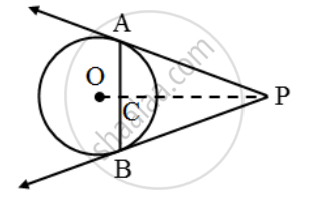Share

Books Shortlist

Prove that the tangents at the extremities of any chord make equal angles with the chord. - CBSE Class 10 - Mathematics

ConceptCircles Examples and Solutions

Question

Prove that the tangents at the extremities of any chord make equal angles with the chord.

Solution

Let AB be a chord of a circle with centre O, and let AP and BP be the tangents at A and B respectively.Suppose the tangents meet at P. Join OP. Suppose OP meets AB at C. We have to prove that ∠PAC = ∠PBC In triangles PCA and PCB, we have

PA = PB

[∵ Tangents from an external point are equal]

∠APC = ∠BPC

[∵PA and PB are equally inclined to OP] and, PC = PC [Common]

So, by SAS – criterion of congruence, we have

∆PAC ≅ ∆PBC

⇒ ∠PAC = ∠PBC

Is there an error in this question or solution?

Video TutorialsVIEW ALL 

Solution Prove that the tangents at the extremities of any chord make equal angles with the chord. Concept: Circles Examples and Solutions.
S